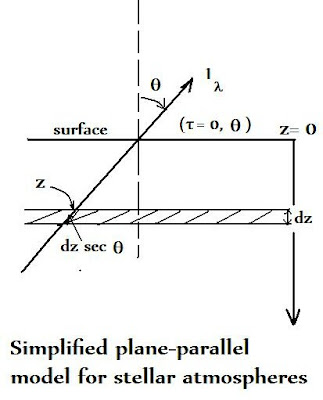## Monday, April 9, 2012

### Simple Solar Radiative Transfer (1)In an earlier blog from two years ago, I examined some basic astrophysics applied to stellar atmospheres, especially the simplified model known as the "gray atmosphere", e.g.

http://brane-space.blogspot.com/2010/10/introducing-some-basic-astrophysics.html

It's now useful to see how we might apply some of this material to solutions of simple solar atmosphere problems. First, a bit of preparatory material-information to supplement that contained in the above link.

a)The flux F_o coming out of the stellar surface is equal to the source function at the optical depth τ = 2/3. This is the important ‘Eddington-Barbier’ relation that paves the way for the understanding of how stellar spectra are formed.

b) Thus, the energy distribution of F_o is that of a black body corresponding to the temperature at an optical depth τ= 2/3. From this, along with some simple substitutions and integrations one finds:

π( F_o ) = σ (Teff)^4 and Teff = T(τ = 2/3)

where σ (= 5.67 x 10^-8 W m^-2 K^-4) is the Stefan-Boltzmann constant. Thus, the temperature at optical depth 2/3 must equal the effective temperature!

c) The appropriate equation of transfer in a simple, plane-parallel atmosphere would be:

dI = j dx - σ I (Θ) dx = (j - σ I (Θ)) dx

or: dI/dx = j - σ I (Θ))

where I (Θ) is the specific intensity

Or, after some further manipulation, and replacing x with τ:

(cos Θ) dI/ dτ = I (Θ) – j/ σ

This is the important equation, in terms of emergent intensity I, that embodies the conservation of radiant energy (i.e. no more radiation can flow out of a star’s surface than can be generated within it and which approaches that surface).

d) To obtain an even more improved basis for calculations entails getting the moments of the intensity, leading to J (mean intensity), H (the “net flux” or the net energy breaching the stellar surface in units of net energy per second per unit area of that surface), and K, the energy density.

The "moments of intensity" are obtained by successive integrations over an element of solid angle - defined as (A/r^2) for a sphere, for example. Thus a sphere with surface area A = 4π r^2 has solid angle (4π r^2 / r^2 ) = 4π steradians. If we are only dealing with a sliver of emergent beam of area 0.01π r^2, say, then the element of solid angle(dw) is:

dw = (0.01π r^2 / r^2 ) = 0.01π sr

Thus: J = 1/4π INT I (Θ) dw

H = 1/4π INT I (Θ)cos Θ dw

K = 1/4π INT I (Θ)cos ^2 Θ dw

e) From the preceding a simplified set of differential equations appears relating H, J, and K, i.e.

dH/ dτ = J - j/ σ

dK/ dτ = H

(Recall, τ is optical depth, not time!)

Or, more simply, 4 πH = const. So, dH/ dτ = J - j/ σ = 0, and the equation of transfer now becomes:

(cos Θ) dI/ dτ = - I + J

e)In using the Eddington approximation (which will apply to the quantities J, H and K), we will also discriminate the radiation intensity I into two components: I1 (in the forward direction) and I2 (in the backward direction). We can then write as follows:

1) J = ½(I1 + I2)

2) H = ¼( I1 - I2)

3) K = J/3

f) Now, we focus on the boundary in Fig. 1, and note that here the optical depth τ= 0, and we must have I2 = 0 also.

Since I2 = 0 then:

H = ¼ I1 and

J = ½I1

so clearly: J = 2H.

Further, K = J/3 or (½I1)/3 so K = Hτ + const.

dK/ dC = H or dK = H dτ and we know τ= 0, hence Hτ + const. on integration.

From this it follows that:

J = H(2 + 3 τ) and K = J/3 = 2H/3

At the boundary everywhere.

And since H = ¼( I1 - I2) = const. then:

I1 (τ) = H(4 + 3τ) and I1 (τ) = 3Hτ

f) A special case occurs if the mean intensity J = B (L), the Planck function, then (since B ~ σT^4/ π):

J = H(2 + 3 τ) = σ T^4/ π

Therefore, the boundary temperature (T_o) approaches the value of the effective (or surface) temperature when τ = 0. So we have the basic relationship:

T_o ^4/ π = 2H

And: σ T^4 = σ T_o ^4/ 2 [a + 3τ]

In the limit of this approximation, Teff ^4 = 2 T_o ^4
And hence:

Teff = (2)^¼ T_o = 1.189 T_o

A Problem:

Consider the solar half-sphere and the energy going into it each second. We know on average photons are absorbed after traveling a distance with optical depth τ =1 in the propagation direction. Averaged over all directions this corresponds to a vertical optical depth of τ= 2/3. Based on this find:

a) The energy going into the half sphere each second

b) The change in (a) over each absorption and re-emission over vertical optical depth.

c) The total absorption and total emission and the relationship between then over all space.

Solution:

(a) This is just: σ(Teff)^4 = π F = 2π S = 2πI

(b) Over a vertical optical depth one has τ = 2/3, then:

2π S/ τ = π F/ (2/3) = 3π F/ 2 or:

S/ τ = 3π F/ 2 (2π) = 3F/4

c) Over all space, the total emission = 4 πS and the total absorption = 4π J, and by radiative equilibrium: 4π S = 4π J so that S = J.